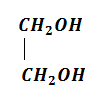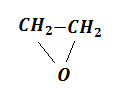n-propyl alcohol and iso-propyl alcohol can be chemically distinguished by which reagent

1. ${\mathrm{PCl}}_{5}$

2. reduction

3. oxidation with potassium dichromate

4. ozonolysis

Concept Questions :-

Alcohols: Preparation, Physical and Chemical Properties
NEET 2021 - Achiever Batch - Aryan Raj Singh

Difficulty Level:

Among the following sets of reactants which one produces anisole?

1. CH3CHO, RMgX

2. C6H5OH, NaOH, CH3I

3. C6H5OH, neutral FeCl3

4. C6H5-CH3, CH3COCl, AlCl3

Concept Questions :-

Ethers: Preparation, Physical & Chemical Properties, Uses
NEET 2021 - Achiever Batch - Aryan Raj Singh

Difficulty Level:

Which one is formed when sodium phenoxide is heated with ethyl iodide?

1. Phenetole

2. Ethyl phenyl alcohol

3. Phenol

4. None of the above

Concept Questions :-

Ethers: Preparation, Physical & Chemical Properties, Uses
NEET 2021 - Achiever Batch - Aryan Raj Singh

Difficulty Level:

Identity Z in the sequence of reactions,

CH3CH2CH=CH2 $\stackrel{\mathrm{HBr}/{\mathrm{H}}_{2}{\mathrm{O}}_{2}}{\to }$Y$\stackrel{{\mathrm{C}}_{2}{\mathrm{H}}_{5}\mathrm{ONa}}{\to }$Z

(a) CH3-(CH2)3-O-CH2CH3

(b) (CH3)2CH2-O-CH2CH3

(c) CH3(CH2)4-O-CH3

(d) CH3CH2-CH(CH3)-O-CH2CH3

Concept Questions :-

Ethers: Preparation, Physical & Chemical Properties, Uses
NEET 2021 - Achiever Batch - Aryan Raj Singh

Difficulty Level:

Reaction of ethyl formate with excess of CH3MgI followed by hydrolysis gives

1. n-propyl alcohol

2. ethanol

3. isopropyl alcohol

4.  propanal

Concept Questions :-

Alcohols: Preparation, Physical and Chemical Properties
NEET 2021 - Achiever Batch - Aryan Raj Singh

Difficulty Level:

In a reaction,

${\mathrm{CH}}_{2}={\mathrm{CH}}_{2}\underset{\mathrm{acid}}{\overset{\mathrm{Hypochlorous}}{\to }}$M$\stackrel{\mathrm{R}}{\to }$M=molecules, R=reagent. M and R are

(a) ${\mathrm{CH}}_{3}{\mathrm{CH}}_{2}\mathrm{Cl}$ and NaOH

(b)  and aq. ${\mathrm{NaHCO}}_{3}$

(c) ${\mathrm{CH}}_{3}{\mathrm{CH}}_{2}\mathrm{OH}$ and HCl

(d)and heat

Concept Questions :-

Alcohols: Preparation, Physical and Chemical Properties
NEET 2021 - Achiever Batch - Aryan Raj Singh

Difficulty Level:

Lucas reagent is

1. conc. HCl and anhy. ZnCl2

2. conc. HNO3 and anhy. ZnCl2

3. conc. HCl and hydrous ZnCl2

4. conc. HNO3 and hydrous ZnCl2

Concept Questions :-

Alcohols: Preparation, Physical and Chemical Properties
NEET 2021 - Achiever Batch - Aryan Raj Singh

Difficulty Level:

The general molecular formula, which represents the homologous series of alkanols is

(1) CnH2nO2

(2) CnH2nO

(3) CnH2n+1O

(4) CnH2n+2O

Concept Questions :-

Alcohols: Preparation, Physical and Chemical Properties
NEET 2021 - Achiever Batch - Aryan Raj Singh

Difficulty Level:

$\mathrm{R}-\mathrm{COOH}\to {\mathrm{RCH}}_{2}\mathrm{OH}$. This reduction cannot be done  by:

(a) ${\mathrm{NaBH}}_{4}$                               (b) Na + alcohol

(c) ${\mathrm{LiAlH}}_{4}$                                (d) all of these

Concept Questions :-

Alcohols: Preparation, Physical and Chemical Properties
NEET 2021 - Achiever Batch - Aryan Raj Singh

Difficulty Level:

What mass of isobutylene is obtained from 37 g of tertiary butyl alcohol by heating with 20% H2SO4 at 363 K, if the yield is 65%?

1. 16 g

2. 18.2 g

3. 20 g

4. 22 g

Concept Questions :-

Alcohols: Preparation, Physical and Chemical Properties